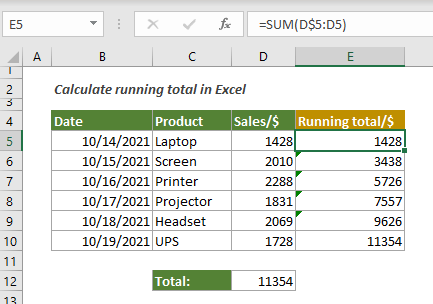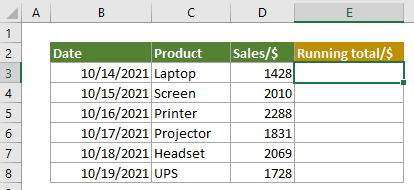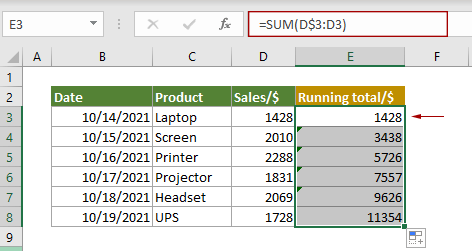## Calculate running total (cumulative sum) in Excel

A running total is also called cumulative sum, which can be used as a metric that tells you what’s the sum of the values so far. In this tutorial, you will learn a simple formula to calculate running total in Excel.#### How to calculate running total in Excel?

Assuming you have a list of date sales data as shown below, calculating the running total tells you what the sales volume was from the first date to a particular date. You can apply the SUM function to easily solve the problem.Generic Formulas

=SUM(A\$1:A1)

Arguments

A\$1: Represents the first cell in the list where you want to calculate the running total. Displays as a row absolute reference.
A1: Represents the first cell in the list where you want to calculate the running total. Displays as a relative reference.

How to use this formula?

1. Select a cell next to the first value in the list.

2. Enter the below formula into it and press Enter key. Select the result cell, drag its AutoFill Handle down to get all running totals in the list.

=SUM(D\$3:D3)Tip: In the formula, clicking the cell reference and then striking the F4 key multiple times helps toggling the absolute and relative references.

Explanation of this formula

=SUM(D\$3:D3)

> The SUM function uses a mixed reference which includes both absolute and relative references to create an expanding range.
> D\$3 – the dollar sign placed before the number means that it has locked the row and this reference will not change when the formula is copied down.

#### Related functions

Excel SUM function
The Excel SUM function adds values.

#### Related formulas

3D sum or sumif across multiple worksheets
This tutorial talks about how to sum the same range of cells across multiple consecutive or discontiguous worksheets in Excel.

Get subtotal by invoice number
This tutorial explains a formula based on the COUNTIF and SUMIF functions to get subtotal by invoice number in Excel.

Subtotal by colors
This step-by-step guide helps you perform subtotal by color with the SUMIF function.

Two-way summary counting
In this tutorial, we will discuss how to use the COUNTIFS function to create two-way summary count in Excel.

### The Best Office Productivity Tools

#### Kutools for Excel - Helps You To Stand Out From Crowd

 Popular Features: Find/Highlight/Identify Duplicates  |  Delete Blank Rows  |  Combine Columns or Cells without Losing Data  |  Round without Formula ... Super VLookup: Multiple Criteria  |  Multiple Value   |  Across Multi-Sheets  |  Fuzzy Lookup... Adv. Drop-down List: Easy Drop Down List  |  Dependent Drop Down List  |  Multi-select Drop Down List... Column Manager: Add a Specific Number of Columns  |  Move Columns  |  Unhide Columns  |  Compare Columns to Select Same & Different Cells ... Featured Features: Grid Focus  |  Design View  |  Big Formula Bar  |  Workbook & Sheet Manager  |  Resource Library (Auto Text)  |  Date Picker  |  Combine Worksheets  |  Encrypt/Decrypt Cells  |  Send Emails by List  |  Super Filter  |  Special Filter (filter bold/italic/strikethrough...) ... Top 15 Toolsets:  12 Text Tools (Add Text, Remove Characters ...)  |  50+ Chart Types (Gantt Chart ...)  |  40+ Practical Formulas (Calculate age based on birthday ...)  |  19 Insertion Tools (Insert QR Code, Insert Picture from Path ...)  |  12 Conversion Tools (Numbers to Words, Currency Conversion ...)  |  7 Merge & Split Tools (Advanced Combine Rows, Split Cells ...)  |  ... and more

Kutools for Excel Boasts Over 300 Features, Ensuring That What You Need is Just A Click Away...

Supports Office/Excel 2007-2021 and 365   |   Available in 44 Languages   |   Easy to Uninstall Completely#### Office Tab - Enable Tabbed Reading and Editing in Microsoft Office (include Excel)

• One second to switch between dozens of open documents!
• Reduce hundreds of mouse clicks for you every day, say goodbye to mouse hand.
• Increases your productivity by 50% when viewing and editing multiple documents.
• Brings Efficient Tabs to Office (include Excel), Just Like Chrome, Edge and Firefox.